# Two runners

Two runners ran simultaneously towards each other from locations distant 34.6 km. The average speed of the first runner was 1/5 higher than the average speed of the second runner.

How long should each ran a 34.6 km, if you know that they meet after 67 minutes?

t1 =  122.8 min
t2 =  147.4 min

### Step-by-step explanation: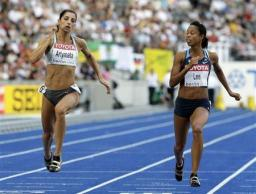Did you find an error or inaccuracy? Feel free to write us. Thank you!Tips to related online calculators
Do you have a linear equation or system of equations and looking for its solution? Or do you have a quadratic equation?
Do you want to convert velocity (speed) units?
Do you want to convert time units like minutes to seconds?

## Related math problems and questions:

• MotionFrom two different locations distant 232 km started against car and bus. The car started at 5:20 with average speed 64 km/h. Bus started at 7:30 with average speed 80 km/h. When they meet? How many kilometers went the bus?
• Against each other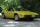From two points A, B distant 23 km at the same time started two cars against each other at speeds 41 km/h and 65 km/h. How long does cars meet and what distance passes each of them?
• Two planes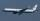Two planes are flying from airports A and B, 420 km distant from each other. The plane from A took off 15 minutes later and flies at an average speed of 40 km/h higher than the plane from B. Determine the average speed of these two aircraft if you know th
• Znojmo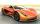From Znojmo to Brno started the truck with a trailer at an average speed 53 km per hour. Against him, 14 minutes later from Brno started the car with an average speed of 1.2-times greater than the truck. How long and how far from Znojmo, they meet if the
• Two trains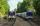Two trains go at each other. First at speed 40 km/h and the second at speed 60 km/h. At what distance will be 30 minutes before they will meet?
• Two citiesThe distance between cities A and B is 132 km. At 9.00 am, the cyclist started the bike at an average speed of 24 km/h, and at 10.00 am started from the B cyclist at an average speed of 30 km/h. How long and far from A will they both meet?
• Runner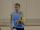Peter ran a speed of 12.4 km / h. After 45 minutes running he had a hiatus. The track is 31 km long. How many kilometers he still to run if he still running at the same speed?
• Two citiesThe car goes from city A to city B at an average speed of 70 km/h, back at an average speed of 50 km/h. If it goes to B and back at an average speed of 60 km/h, the whole ride will take 8 minutes less. What is the distance between cities A and B?
• Two carsTwo cars (trucks and passenger) drove simultaneously from two places 50 km away. If they go against each other, they will meet in 30 minutes. If they follow each other in the same direction, the car will catch up with the truck in 2.5 hours. What is the s
• Two cars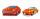From the town A to town B started two cars. The first at 7:00 at average speed 60 km per hour, the second at 10:00 at average speed 100 km per hour. The first car will not stay in B, and on the way back meet the second car at half way from A to B. At what
• Motion 2At 10 o'clock started from place A car speed at 90 km/h. Twenty minutes after starting another car from place B at a speed 60 km/h . Distance places A and B is 120 kilometers. What time and where do they meet?
• Average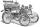What is the average speed of the car, where half of the distance covered passed at speed 66 km/h and the other half at 86 km/h.
• Average speed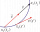The car drove on one section of the highway for half an hour at a speed of 80 km/h. Then he reduced his speed to 60km/h. It went three-quarters of an hour at a speed of 60 km/h. What was the average speed of the car?
• CarThe driver of a car is to get to 608 km distant city. From atlas found that 162 km will have to pass through the cities at an average speed 48 km/h. The remainder of the journey passes outside the cities at average speed 116 km/h. Calculate how many hours
• Two friendsTwo friends on a bicycle rode against each other from two places 49 km apart. The first set off at 8.00 at a speed of 20km/h, the second 12 minutes later at a speed of 25km/h. What time do they meet? How many km will each of them travel since then?
• Pedestrian and the cyclist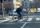Pedestrian and cyclist went against each from the two cities that are distant 40 km. Pedestrian started 30 minutes before the cyclist and walk an average speed of 5 km/h. What speed went cyclist when met after 120 minutes from the start of the pedestrian?
• Blackjack TU160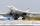Bomber Tupolev TU160 started at 9:00 from the airport and flew at average speed of 1850 km/h toward the airfield B distant 3500 km. After evaluating the danger from the airport B started at 9:45 B towards A fighter MIG 29 at an average speed 2300 km/h. At